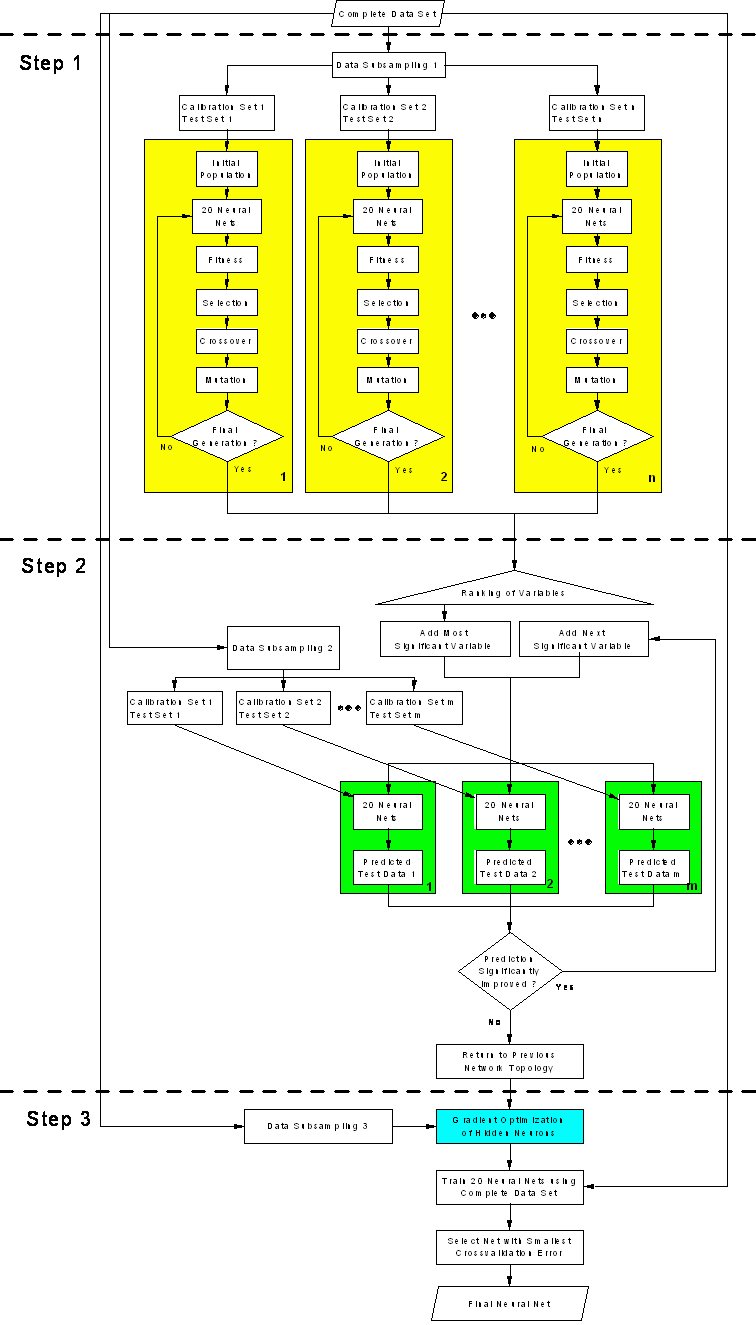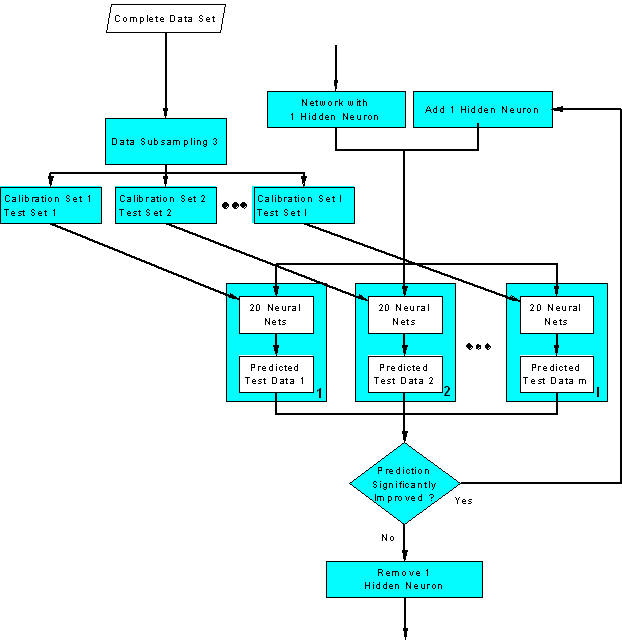Home News About Me Ph. D. Thesis Abstract Table of Contents 1. Introduction 2. Theory  Fundamentals of the Multivariate Data Analysis 3. Theory  Quantification of the Refrigerants R22 and R134a: Part I 4. Experiments, Setups and Data Sets 5. Results  Kinetic Measurements 6. Results  Multivariate Calibrations 7. Results  Genetic Algorithm Framework 7.1. Single Run Genetic Algorithm 7.2. Genetic Algorithm Framework - Theory 7.3. Genetic Algorithm Framework - Results 7.4. Genetic Algorithm Framework  Conclusions 8. Results  Growing Neural Network Framework 9. Results  All Data Sets 10. Results  Various Aspects of the Frameworks and Measurements 11. Summary and Outlook 12. References 13. Acknowledgements Publications Research Tutorials Downloads and Links Contact Search Site Map Print this Page## 7.2.   Genetic Algorithm Framework - Theory

In recent literature, several approaches are reported to solve the different problems of single runs of GA:

1.      Massart and Leardi , use a very refined algorithm for the variable selection, which is based on parallel runs of many GA with different combinations of test and calibration data. Then a validation step is performed to find the best variable subset. The GA is a hybrid algorithm using a stepwise backward elimination of variables to find the smallest possible subset of variables. Although this approach is very promising, Jouan-Rimbau et al.  showed that this algorithm is still partly subject to chance correlation.

2.      In  Leardi et al. use 100 runs of GA with the same calibration and test data sets. The final model is obtained by adding systematically the variables, which are ranked according to the frequency of selection of the GA runs and by using the combination with the smallest error of prediction. In  this algorithm is modified by the different GA runs learning from each other.

3.      In  the predictions are averaged by several models found by different GA runs. Yet, the average prediction was not better than the prediction by a single model.

4.      In  10 runs of GA are performed by using different calibration and test data subsets. The final model uses all variables, which were selected at least 5 times, whereby this limit is rather arbitrary.

The genetic algorithm framework proposed in this work picks several elements of the studies mentioned above and is presented in the flow diagram in figure 44. The framework can be divided into three steps. The first step consists of multiple parallel runs of the GA presented in section 2.8.9 and in section 7.1 using different calibration and test data subsets (yellow boxes in the flow diagram). Variables, which are represented higher than average in the final population of each GA run, are collected over all GA runs and are ranked according to the frequency of appearance in the final populations. The second step of the framework finally selects the variables in an iterative procedure by adding the variables to the neural network model according to their rank in a stepwise procedure. The neural network is evaluated by the use of different calibration and test data subsets (green boxes in figure 44). The RMSE of prediction of all test data sets is compared with the RMSE of the previous model. If the RMSE is lower (see section 10.2), the last variable is accepted and the procedure is repeated adding the next important variable until the predictions are not improved any more.

In the third step, the final neural network topology is determined. First, the number of hidden neurons of a single hidden layer is optimized in an iterative procedure, which is shown in figure 45. Starting with fully connected neural networks with 1 hidden neuron additional fully connected neurons are added until the error of prediction of the test data doesn't improve any more, whereby the l different test data subsets are generated by a data subsampling procedure. Finally, this neural network topology is trained with the complete data set several times, and the neural net with the smallest error of crossvalidation should be used as final optimized model and should be validated by an external data set not used during the complete variable selection algorithm.

In all three major steps of the framework, the complete data set is split several times into a calibration (75 %) and a test (25 %) subset, which was done by a random sub­sampling procedure (see section 2.4) resulting in rather pessimistic predictions of the test data. Conse­quently, according to expression (16) models are preferred, which are more predictive and which yield a better interpolation.

As already stated in section 2.8.5, the choice of a in the fitness function (16) influences the numbers of variables being selected during each run of a GA. A too high value of a ignores partly the accuracy of the neural nets and ends in only few variables being selected. Consequently, there might be too few variables selected in the first step to be added to the neural net in the second step. This problem can be recognized by all variables with a ranking higher than "0" being used for the neural net in the second step. On the other side, a too low value of a results in too many variables being selected. This can be detected by the absence of a differentiation of the variables in the ranking. An empirical way to select an optimal a is based on running a single GA with different values of a and on choosing that a, which results in the selection of the number of variables expected to be needed for the calibration. A good choice to start with is setting a to "1" for these single runs of the GA. Yet, preliminary studies showed that the parallel runs of the GA make the framework quite robust towards the choice of a and to the population size, which is suggested to be set to the number of variables to select from. Although the framework seems to be complex on the first sight, this robustness renders the algorithm quite user-friendly.figure 44:  Flow chart of the genetic algorithm framework.figure 45:  Optimization of the number of hidden neurons. This figure is a detailed flow chart of the blue box of the genetic algorithm framework shown in figure 44.

 Page 103 © Frank Dieterle, 03.03.2019